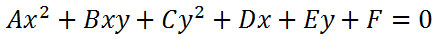Hyperbolas
 Home > Lessons > Conic Sections Search | Updated May 28th, 2020
Introduction

In this section, you will learn how to graph hyperbolas. Here is a list of the sections within this webpage:

 How to Graph a Hyperbola The instructional video below will demonstrate how to graph a hyperbola. It details how to locate all critical points, which includes the center, two vertices, and two foci. It also shows how to obtain the equations of the asymptotes, which are used to sketch the hyperbola.ideo: Graphing Hyperbolasuiz: Graphing Hyperbolasuiz: Equations of Hyperbolas Identifying Conic Sections in General Form We have seen equations of conic sections in standard form. Now we will look at equations of conic sections in general form. The general form of a conic section looks like this.To be able to identify these equations of conic sections in general form, we will make use of a graphic that will help us. The graphic below is called a process flow.It is called a process flow because the graphic informs us to start at the top and move our way down it. The steps within each level tell us what to do and all we have to do is answer a few questions.     To demonstrate how this process flow can be used, follow the example below. Example:We will work our way down the process flow by starting with level one. It asks, "Is there only one squared term?" Let us examine the equation to answer the question.Since our problem has two squared terms, we have to say "no." So, it is not a parabola and we have to go on to level two as directed.     Level two asks, "Are the coefficients of the squared terms equal?" Again, we need to look at the equation to answer.After reviewing the coefficients, we have to say they are not equal. 9 is not equal to -16. So, it's not a circle and we have to proceed to level 3, as directed by the graphic.     Level three asks, "Are the coefficients of the squared terms opposite in sign?" Let's examine the graphic again.The answer is "yes." 9 is positive and -16 is negative. They do have opposite signs. They are not opposite in value, but they are opposite in that one is positive and the other is negative. According to the process flow, the conic section is a hyperbola.     It is clear that it is necessary to know basic terminology to use this process flow. Phrases like squared term, coefficient, and opposite sign need to be fully grasped to benefit by the graphic.     Test your ability to use this process flow. Make use of this quiz.uiz: Identify the Conic Section Videos Here are videos related to the lessons above.ideo: Graphing Hyperbolas
 Quizmasters After reading the lessons, try our quizmasters. MATHguide has developed numerous testing and checking programs to solidify these skills:uiz: Graphing Hyperbolasuiz: Equations of Hyperbolas Activities Here are activities related to the lessons above.ctivity: Hyperbolasctivity: Conics: Supplemental Related Lessons Here related lessons to view.esson: Circlesesson: Ellipsesesson: Parabolasesson: Conic Sectionsesson: Graphing Linesesson: The Point-Slope Formulaesson: Graphing Linear Inequalitiesesson: Flight of a Projectileesson: Completing the Square